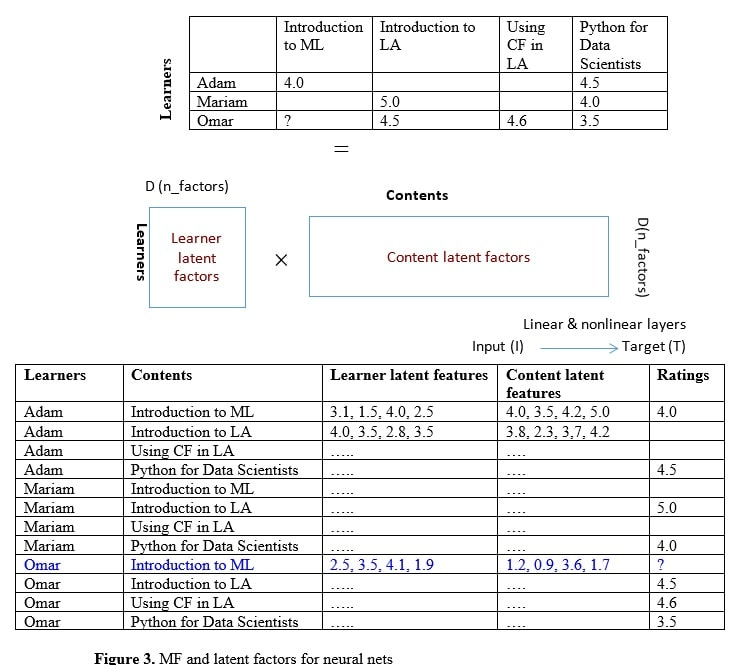# Neural Nets CF

## Main Charateristics of Neural Nets Aproach

The clustering based algorithm (KNN) follows the same approach as the memory-based model; however, the similarities between learners are measured based on an unsupervised learning algorithm.

One of the neural nets model is an extension of the MF method. The SVD and PCA methods decompose the original matrix into a product of two orthogonal matrices but for neural nets the matrices do not have to be orthogonal.

The neural nets model will have to learn the values of the latent factor matrix. The learner hidden features and content hidden features are looked up from the latent factor matrices for specific content-learner combination, which represent input values for additional linear and non-linear layers.

In addition, this input could also be given to an activation function rectifier linear unit (ReLu), linear/sigmoid layers, and then learn the corresponding weights using stochastic gradient descent optimization algorithms. Figure 3 uses the example in figure 2 (already discussed in the matrix factorization section) and shows how neural nets method could be used.